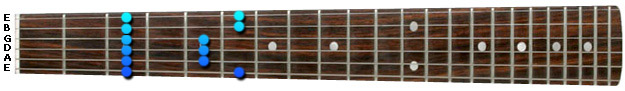• Lessons
• Articles
Tutorial - Pentatonic Scales

# Pentatonic Scales - Position 1

Sean Conklin 456 lessons
Tutorial

## Pentatonic Scales

With each scale position, we are going to play the pattern with eighth notes, then triplets, then sixteenth notes. The fingering you use is optional. Since these scales are 2-notes-per-string, I have a personal preference of just using two fingers: my index finger and my ring finger. However, I'd encourage you to use all four of your fingers if you can.

Here we have the 1st position of the G minor pentatonic scale. Out of the five positions of the pentatonic scale, this is the position that primarily represents the minor key. Keep in mind that the very first note in this position is the root note. So when you're looking to play the pentatonic scale in a minor key, you can simply assign the first position to the appropriate root note on the Low E string.

For example, let's say I told you that I want you to solo in the key of B minor. What would you do? You could simply find the B note on the Low E string, then apply your pentatonic position 1 to that note. So in the case of B minor, you'd simply play the 1st position starting on the 7th fret of the Low E string. However, in this lesson we are in G minor, so notice how the first position is starting on the 3rd fret Low E string (the G note).

Position 1Send this to a friend